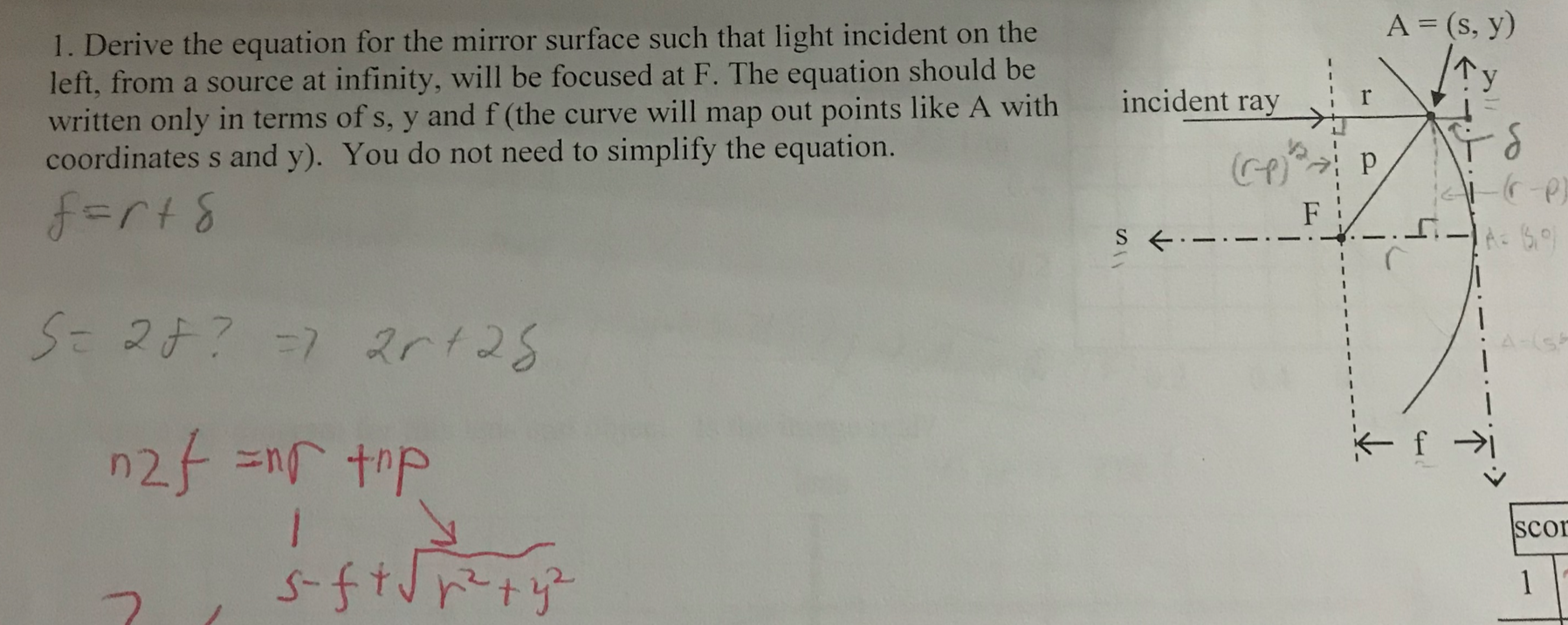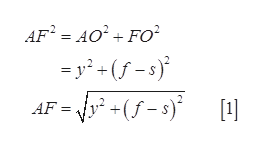# A = (s, y)1. Derive the equation for the mirror surface such that light incident on theleft, from a source at infinity, will be focused at F. The equation should bewritten only in terms of s, y and f (the curve will map out points like A withcoordinates s and y). You do not need to simplify the equation.Cyincident rayf=rt8S=2¢? =7 2rt28A-(sn2f =nr topscorsftJpetyz1

Question
18 viewshelp_outlineImage TranscriptioncloseA = (s, y) 1. Derive the equation for the mirror surface such that light incident on the left, from a source at infinity, will be focused at F. The equation should be written only in terms of s, y and f (the curve will map out points like A with coordinates s and y). You do not need to simplify the equation. Cy incident ray f=rt8 S=2¢? =7 2rt28 A-(s n2f =nr top scor sftJpetyz 1 fullscreen
check_circle

Step 1

The figure shows the light incident from a source at infinity.

Step 2

Using the Pythagoras theorem in triangle AFO.help_outlineImage TranscriptioncloseAF' = AO' + FO = y* + (f - s)° AF = y* +(f - s)*  fullscreen
Step 3

According to the law of reflection, the angle of in...

### Want to see the full answer?

See Solution

#### Want to see this answer and more?

Solutions are written by subject experts who are available 24/7. Questions are typically answered within 1 hour.*

See Solution
*Response times may vary by subject and question.
Tagged in

### Science